## CBSE Previous Year Question Papers Class 12 Physics 2016 Delhi

Section – A

Question 1.
A point charge +Q is placed at point O as shown in the figure. Is the potential difference VA – VB positive, negative or zero ?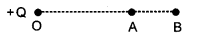Potential at a distance r from a given point charge Q is given by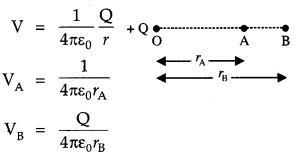Question 2.
How does the electric flux due to a point charge enclosed by a spherical Gaussian surface get affected when its radius is increased ? 
According to Gauss’s law
$$\phi=\int \overrightarrow{\mathrm{E}} \cdot \overrightarrow{d s}=\frac{q_{e n}}{\varepsilon_{0}}$$

Flux depends only on the charge enclosed and not on the radius. Hence, the electric flux remains constant.

Question 3.
Write the underlying principle of a moving coil galvanometer. 
When a current carrying coil is placed in magnetic field then it experiences a torque.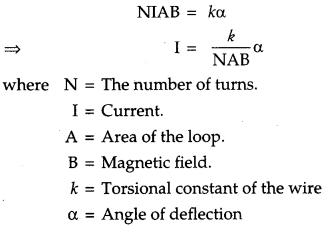Question 4.
Why are microwaves considered suitable for radar systems used in aircraft navigation ? 
Microwaves are considered suitable because they have a short wavelength range they are suitable for radar system used in aircraft navigation.

Question 5.
Define ‘quality factor’ of resonance in series LCR circuit. What is its SI unit ? 
The Q factor of series resonance circuit is defined as the ratio of the voltage developed across the inductor or capacitor at resonance to the impressed voltage, which is the voltage across R.
$$Q=\frac{1}{R} \sqrt{\frac{L}{C}}$$
It is dimensionless quantity. Hence, it has no units.

Section-B

Question 6.
Explain the terms
(i) Attenuation and
(ii) Demodulation used in Communication System. 
(i) Attenuation : The loss of strength of a signal while propagating through a medium is known as attenuation.
(ii) Demodulation : The process of retrieval of information from the carrier wave at the receiver end is termed as demodulation. This is the reverse process of modulation.

Question 7.
Plot a graph showing variation of de-Broglie wavelength λ versus 1/√v, where V is accelerating potential for two particles A and B carrying same charge but of masses m1, m2 (m1 > m2). Which one of the two represents a particle of smaller mass and why ? 
We know that,Hence, the particle with lower mass (m2) will have greater slope.

Question 8.
A nucleus with mass number A = 240 and BE/A = 7.6 MeV breaks into two fragments each of A = 120 with BE/A = 8.5 MeV. Calculate the released energy. 
OR
Calculate the energy in fusion reaction: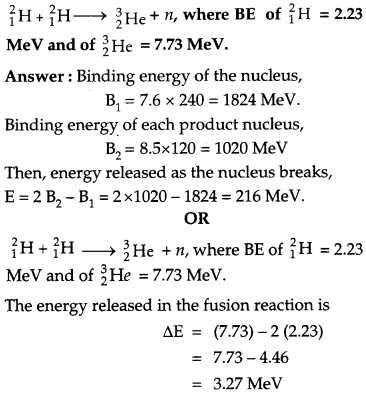Question 9.
Two cells of emfs 1.5 V and 2.0 V having internal resistances 0.2 Ω, and 0.3 Ω, respectively are connected in parallel. Calculate the emf and internal resistance of the equivalent cell.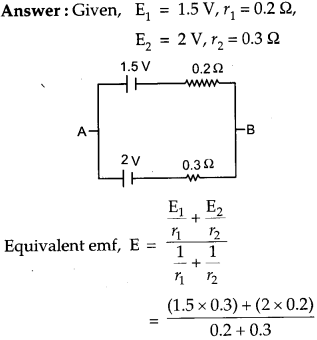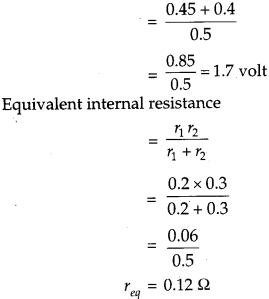Question 10.
State Brewster’s law.
The value of Brewster angle for a transparent medium is different for light of different colors. Give reason. 
Brewster’s law: The law states that the tangent of the polarizing angle of incidence for a given medium is equal to the refractive index of the medium. The light incident at this angle when reflects back is perfectly polarized,
i.e.  μ = tan ip
The refractive index of a material depends on the color or wavelength of light. As the polarizing angle depends on refractive index (p = tan ip), so it also depends on wavelength of light.

Section-C

Question 11.
A charge is distributed uniformly over a ring of radius ‘a’. Obtain an expression for the electric intensity E at a point on the axis of the ring. Hence show that for points at large distances from the ring, it behaves like a point charge. 
Suppose we have a ring of radius a that carries a uniformly distributed positive charge q.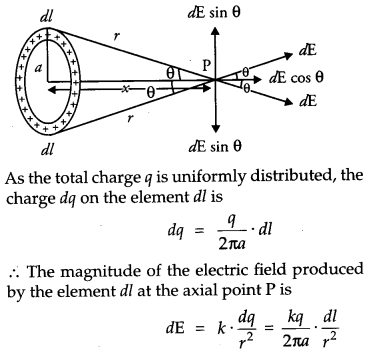The electric field dE has two components.
(i) The axial components dE cos θ and
(ii) The perpendicular component dE sin θ.
Since the perpendicular component of any two diametrically opposite elements are equal and opposite, they cancel out in pairs. Only the axial components will add up to produce the resultant field.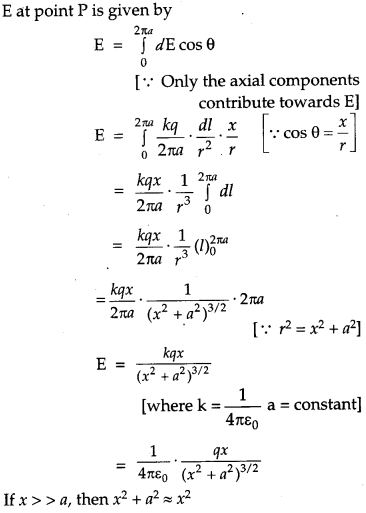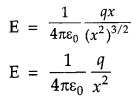This expression  is similar to electric field due to a point charge

Question 12.
Write three characteristic features in photo electric effect which cannot be explained on the basis of wave theory of light, but can be explained only using Einstein’s equation. 

• Existence of threshold frequency : According to wave theory, there should not exist any threshold frequency but Einstein’s theory explains the existence of threshold frequency.
• Dependence of kinetic energy on frequency of incident light: According to wave theory, the maximum kinetic energy of emitted electrons should depend on intensity of incident light and not on frequency whereas Einstein’s equation explains that it dependence on frequency and not on intensity of the incident light.
• Instantaneous emission of electrons : According to wave theory there should be time lag between emission of electrons and incident of light whereas Einstein’s equation explains why there is no time lag between incident of light and emission of electrons.

Question 13.
(a) Write the expression for the magnetic force acting on a charged particle moving with velocity v in the presence of magnetic field B.
(b) A neutron, an electron and an alpha particle moving with equal velocities, enter a uniform magnetic field going into the plane of the paper as shown. Trace their paths in the field and justify your answer.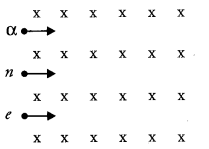(a) A charge particle having charge q is moving with velocity V in a magnetic field of field strength ‘B’ then the force acting on it is given by the formula $$F=q(\vec{\nu} \times \vec{B})$$ and F = qv B sin θ (where θ is the angle between velocity vector and magnetic field).
Direction of force is given by the cross product of velocity and magnetic field.
(b)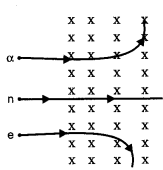α particle will trace circular path in anticlockwise direction as it’s deviation will be in the direction
of  $$(\vec{v} \times \vec{B})$$
Neutron will pass without any deviation as magnetic field does not exert and force on neutral particle. Electron will trace circular path in clockwise direction as its deviation will be in the direction opposite to $$(\vec{v} \times \vec{B})$$ with a smaller radius due to large  charge/mass ratio as $$r=\frac{m v}{q \mathrm{B}}$$

Question 14.
(a) Define mutual inductance.
(b) A pair of adjacent coils has a mutual inductance of 1.5 H. If the current in one coil changes from 0 to 20 A in 0.5 s, what is the change of flux linkage with the other coil ? 
(a) Mutual induction is the phenomenon of production of induced emf in one coil due to change of current or flux in the neighboring coil. The coil in which the current changes is called primary coil and the coil in which emf is induced is called the secondary coil.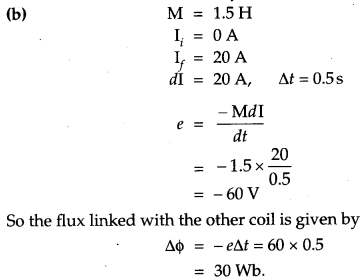Question 15.
Two parallel plate capacitors X and Y have the same area of plates and same separation between them. X has air between the plates while Y contains a dielectric medium of ∈r = 4.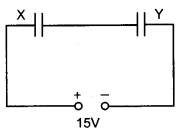(i) Calculate capacitance of each capacitor if equivalent capacitance of the combination is 4 μF.
(ii) Calculate the potential difference between the plates of X and Y.
(iii) Estimate the ratio of electrostatic energy stored in X and Y.
(i) Let capacitance of X be C1 and capacitance of Y be C2.Question 16.
Two long straight parallel conductors carry steady current I1 and I1 separated by a distance d. If the currents are flowing in the same direction, show how the magnetic field set up if one produces an attractive force on the other. Obtain the expression for this force. Hence define one ampere. 
Magnetic field produced on the wire (carrying current I2) due to I1 will be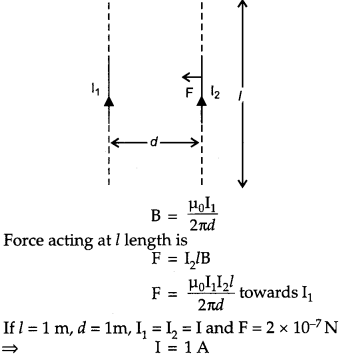So one ampere is defined as the current, which when maintained in two parallel infinite length conductors, held at a separation of one meter will produce a force of 2 x 10-7 N per meter on each conductor.

Question 17.
How are e.m. waves produced by oscillating charges ?
Draw a sketch of linearly polarized e.m. waves propagating in the Z-direction. Indicate the directions of the oscillating electric and magnetic fields. 
OR
Write Maxwell’s generalization of Ampere’s circuital law. Show that in the process of charging a capacitor, the current produced within the plates of the capacitor is $$\i=\varepsilon_{0} \frac{d \phi_{\mathrm{E}}}{d t}$$
where ΦE is the electric flux produced during charging of the capacitor plates.
A charge oscillating with some frequency, produces an oscillating electric field in space, which in turn produces an oscillating magnetic field perpendicular to the electric field. This process goes on repeating, producing e.m. waves in space perpendicular to both the fields.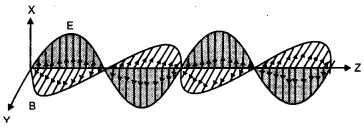The direction of electric and magnetic fields are perpendicular to each other and are also perpendicular to the direction of propagation of the wave.
OR
Correction in Ampere’s circuital law (Modified Ampere’s law) : Maxwell removed the problem of current continuity and inconsistency observed in Ampere’s circuital law by introducing the concept of displacement current. Displacement current arises due to change in electric flux with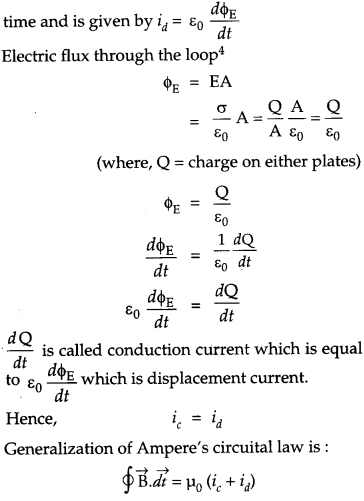Conduction current is due to the flow of charges but displacement current is not because of the flow of charges but it is due to the change in electric flux.

Question 18.
(a) Explain any two factors which justify the need of modulating a low frequency signal,
(b) Write two advantages of frequency modulation over amplitude modulation. 
(a) 1. Size of Antenna : The size of antenna required will be of order of λ /4. When frequency is small, the height of antenna should be large. So audio frequency signal should be modulated over a high frequency carrier wave to increase its frequency.
2. Effective power radiated by an Antenna : As power radiated $$\propto \frac{1}{\lambda^{2}}$$ hence when frequency is As increased then the power radiated will be more,

(b) Advantages of frequency modulation over amplitude modulation:

• Noise can be reduced.
• Transmission efficiency is more because the amplitude of an FM wave is constant.

Question 19.
(a) Write the functions of three segments of a transistor.
(b) Draw the circuit diagram for studying the input and output characteristics of n-p-n transistor in common emitter configuration. Using the circuit, explain how input, output characteristics are obtained.  

Question 20.
(a) Calculate the distance of an object of height h from a concave mirror of radius of curvature 20 cm, so as to obtain a real image of magnification 2. Find the location of image also.
(b) Using mirror formula, explain why does a convex mirror always produce a virtual image.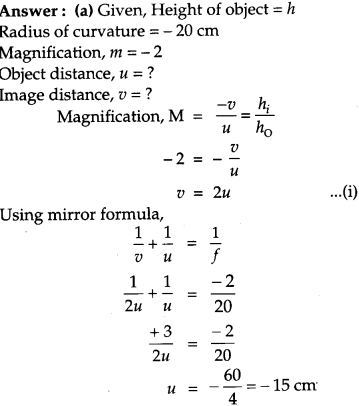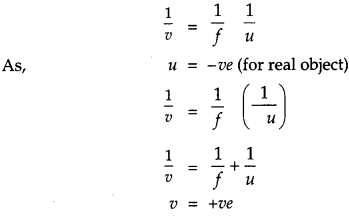Hence, it will form  virtual and erect image.

Question 21.
(a) State Bohr’s quantization condition for defining stationary orbits. How does de- Broglie hypothesis explain the stationary orbits ?
(b) Find the relation between the three wavelengths λ1 λ2 and λ3 from the energy level diagram shown below.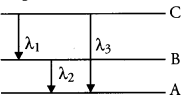(a) Quantization condition : Of all possible circular orbits allowed by the classical theory, the electrons are permitted to circulate only in those orbits in which the angular momentum of an electron is an integral multiple of h/2π ; h being planck constant.
therefore, for any permitted  orbit,
$$\mathrm{L}=m v r=\frac{n h}{2 \pi} ; n=1,2,3, \ldots \ldots$$

Where L, m, and v are the angular momentum, mass and speed of the electron respectively, r is the radius of the permitted orbit and n is positive integer called principle quantum number.

The above equation is Bohr’s famous quantum condition. When an electron of mass m is confined to move in a line of length l with velocity v, the de-Broglie wavelength λ , associated with electron is: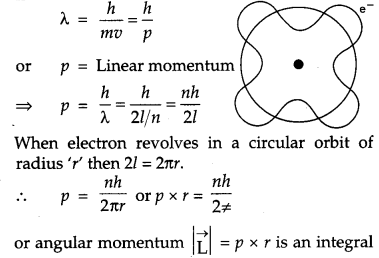multiple of h/2π, which is Bohr’s quantisation of angular momentum.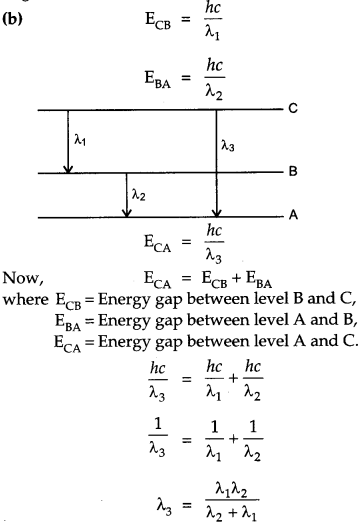Question 22.
Draw a schematic ray diagram of reflecting telescope showing how rays coming from a distant object are received at the eye-piece. Write its two important advantages over a refracting telescope. 
Reflecting Telescope : The reflecting telescope make use of a concave mirror as objective. The rays of light coming from distant object are incident on the objective (parabolic reflector). After reflection the rays of light meet at a point where another convex mirror is placed. This mirror focuses light inside the telescope tube. The final image is seen through the eye¬piece. The images produced by the reflecting telescope is very bright and its resolving power is high.(i) The resolving power (the ability to observe two object distinctly) is high, due to the large diameter of the objective.
(ii) There is no chromatic aberration as the object is a mirror.

Section – D

Question 23.
Meeta’s father was driving her to the school. At the traffic signal she noticed that each traffic light was made of many tiny lights instead of a single bulb. When Meeta asked this question to her father, he explained the reason for this. Answer the following questions based on above information:
(i) What were the values displayed by Meeta and her father?
(ii) What answer did Meeta’s father give ?
(iii) What are the tiny lights in traffic signals called and how do these operate ? 
(ii) Meeta’s father said that these are LED light which consume less power and have high reliability.
(iii) The tiny lights in traffic signals are Light Emitting Diode. These are operated by connecting the p-n junction diode in forward biased condition

Section-E

Question 24.
(a) An a.c. source of voltage V = V0 sin ωt is connected to a series combination of L, C and R. Use the phasor diagram to obtain expressions for impedance of the circuit and phase angle between voltage and current. Find the condition when current will be in phase with the voltage. What is the circuit in this condition called ?
(b) In a series LR circuit XL = R and power factor of the circuit is P1 When capacitor with capacitance C such that XL = Xc, is put in series, the power factor becomes P2. Calculate P1/P2. 
OR
(a) Write the function of a transformer. State its principle of working with the help of a diagram. Mention various energy losses in this device.
(b) The primary coil of an ideal step up transformer has 100 turns and transformation ratio is also 100. The input voltage and power are respectively 220 V and 1100 W.
Calculate:
(i) number of turns in secondary.
(ii) current in primary.
(iii) voltage across secondary.
(iv) current in secondary
(v) power in secondary.
(a) Let a series LCR circuit is connected to an a.c. source V (Fig). We take the voltage of the source to be V = V0 sin ωt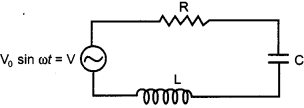The a.c. current in each element is the same at any time, having the same amplitude and phase. It is given by,
I = I0 sin (ωt + Φ)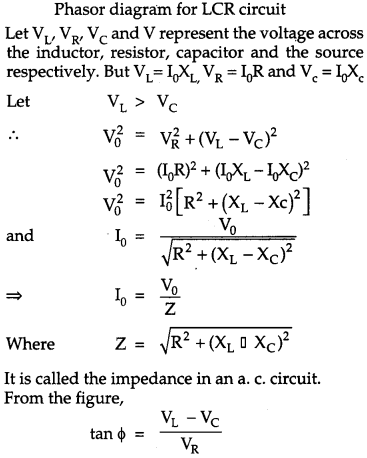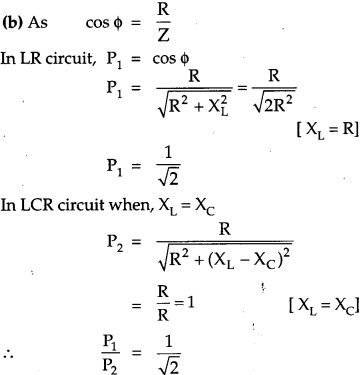OR
(a) A transformer is an electrical device for converting an alternating current at low voltage into high voltage or vice-versa.
1. If it increases the input a.c. voltage and decreases the, it is called step up transformer.
2. If it decreases the input a.c. voltage and increases the current, it is called step down transformer.
Principle : It works on the principle of mutual induction i.e., when a changing current or flux is passed through one of the two inductively coupled coils, an induced emf is set up in the other coil.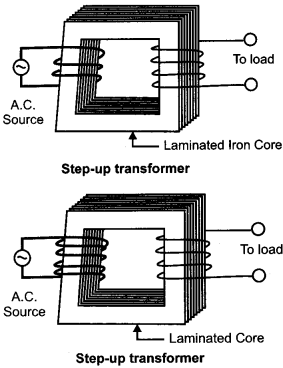Working Theory : As the a.c. flows through the primary, it generate an alternating magnetic flux in the core which passes through the secondary coil

Let N1 = No. of turns in primary coils
N2 = No. of turns in secondary coils
This changing flux set up an induced emf in the secondary, also a self induced emf in the primary.

If there is no leakage of magnetic flux, then flux linked with each turn of the primary will be equal to that linked with each of the secondary. According to Faraday’s law of induction

Induced emf in the primary coil, $$\varepsilon_{1}=-N_{1} \frac{d \phi}{d t}$$
Induced emf in the secondary coil, $$\mathrm{e}_{2}=-\mathrm{N}_{2} \frac{d \phi}{d t}$$
Where, $$\frac{d \phi}{d t}=$$ = Rate of change of magnetic flux associated with each turn.
Φ = Magnetic flux linked with each turn of the primary or secondary at any instant.
$$\frac{\varepsilon_{2}}{\varepsilon_{1}}=\frac{N_{2}}{N_{1}}$$

Energy losses in. transformer :

• Copper loss : Some energy is lost due to the heating of copper wires used in the primary and secondary winding’s. This power loss (P = I2R) can be minimized by using thick copper wires of low resistance.
• Eddy current loss or Iron loss: The alternating , magnetic flux induces eddy current in their on core which leads to some energy loss in the form of heat. This loss can be reduced by using laminated iron core.
• Hysteresis loss : When the iron core is subjected to a cycle of magnetization the core gets heated up due to hysteresis, having low hysteresis loop.
• Flux leakage : The magnetic flux produced by the primary may not fully pass through the secondary. Some of the flux may leak into air. This loss can be minimized by winding the primary and secondary coils over one another.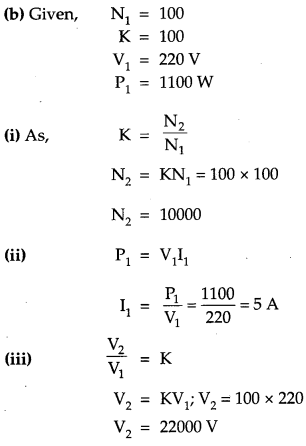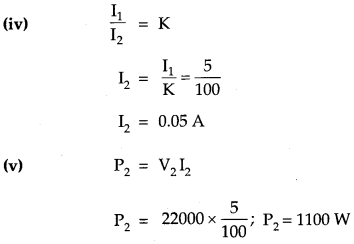Question 25.
(a) In Young’s double slit experiment, deduce the condition for
(i) constructive, and
(ii) destructive interference at a point on the screen. Draw a graph showing variation of intensity in the interference pattern against positionon ‘x’ the screen.
(b) Compare the interference pattern observed in Young’s double slit experiment with single slit diffraction pattern, pointing out three distinguishing features. 
OR
(a) Plot a graph to show variation of the angle of deviation as a function of angle of incidence for light passing through a prism. Derive an expression for refractive index of the prism in terms of angle of minimum deviation and angle of prism.
(b) What is dispersion of light ? What is its cause ?
(c) A ray of light incident normally on one face of a right isosceles prism is totally reflected as shown in fig. What must be the minimum value of refractive index of glass? Give relevant calculations.(a) Let the two waves arising from the slits A and B have the amplitudes a and b and the phase difference Φ. Such that y1=a sin ωt and y2 = b sin (ωt + Φ).

The resultant displacement is given as :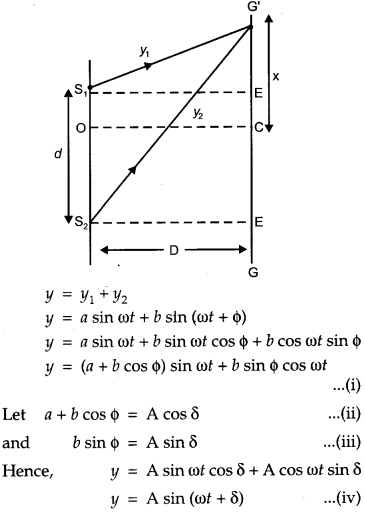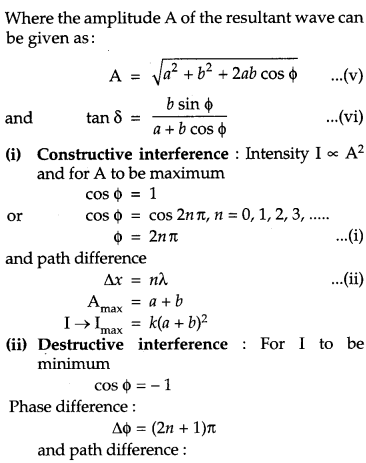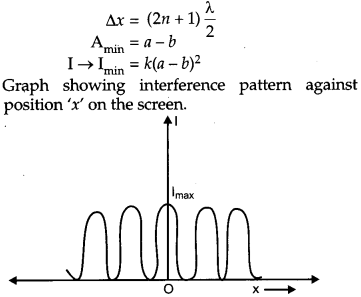b) Comparison of interference pattern observed in Young’s double slits and the single slit diffraction:

 Interference Diffraction 1. Interference is the result of superposition of secondary waves starting from two different wave fronts originating from two coherent sources. 1. Diffraction is the result of superposition of secondary waves starting from different part of same wave front 2. All bright and dark fringes are of equal width 2. The width of central bright fringe is twice the width of any secondary maximum 3. All bright fringes are of same intensity 3. Intensity of bright fringes decreases as we move away from central bright fringes on either side.

OR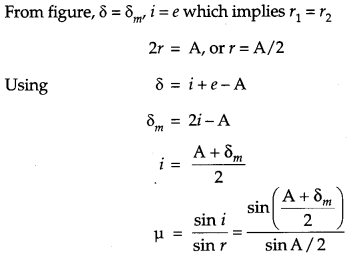Dispersion. of light : Dispersion is often observed as light passes through a triangular prism. Upon passing through the prism, the white light is separated into its component colours : red, orange, yellow, green, blue, and voilet. The separation of visible light into its different colours is known as dispersion. Dispersion occurs because for different colour of light a transparent medium will have different refractive indices (μ). as different colours have different speed in transparent medium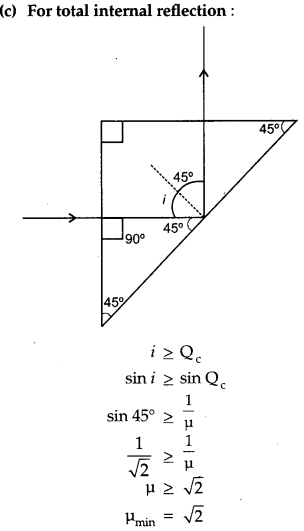Question 26.
(a) Define the term drift velocity.
(b) On the basis of electron drift, derive an expression for resistivity of a conductor in terms of number density of free electrons and relaxation time. On what factors does resistivity of a conductor depend ?
(c) Why alloys like constantan and manganin are used for making standard resistors ? 
OR
(a) State the principle of working of a potentiometer.
(b) In the following potentiometer circuit AB is a uniform wire of length 1 m and resistance 10 Ω. Calculate the potential gradient along the wire and balance length AO (= l).(a) Drift velocity is defined as the average velocity with which the free electrons are drifted towards the positive terminal under the effect of applied electric field. Thermal velocities are randomly distributed and average thermal velocity is zero.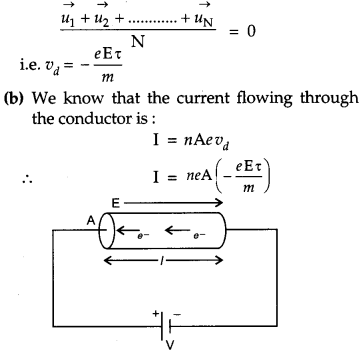Where ρ is the specific resistance or resistivity of the material of the wire. It depends on number of free electron per unit volume and temperature.
(c) They are used to make standard resistors because:
1. They have high value of resistivity.
2. Temperature coefficient of resistance is less.
3. They are least affected by temperature.
OR
(a) Principle of potentiometer : The basic principle of potentiometer is that when a constant current flows through a wire of uniform cross-section area then the potential drop across any length of the wire is directly proportional to that length.
A potentiometer is a device used to measure an unknown emf or potential difference and internal resistance of a cell accuratly.